Скачать презентацию Solving Proportions Warm Up Problem of the Day

• Количество слайдов: 26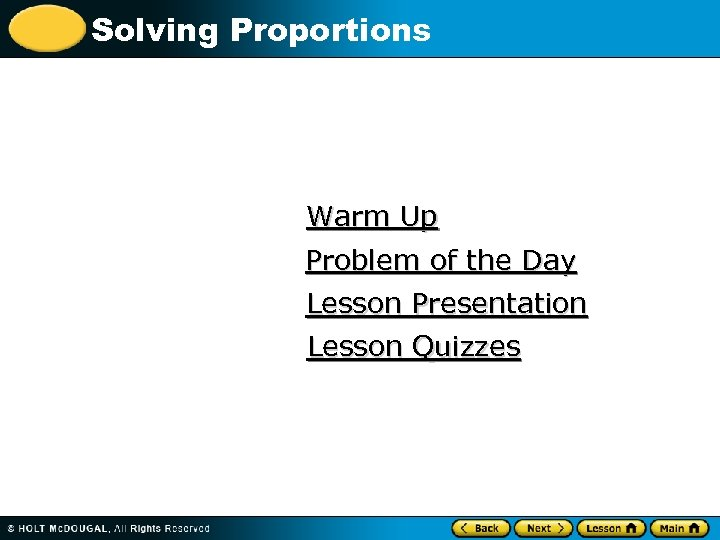Solving Proportions Warm Up Problem of the Day Lesson Presentation Lesson Quizzes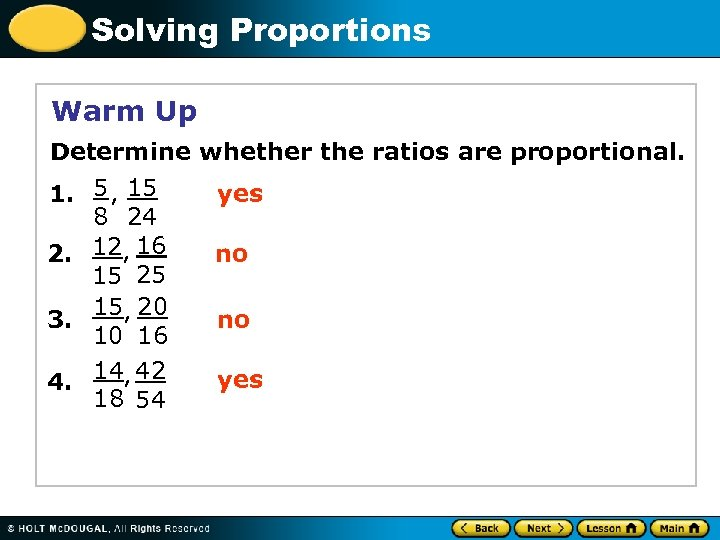Solving Proportions Warm Up Determine whether the ratios are proportional. 1. 5 , 15 8 24 2. 12, 16 15 25 15, 20 3. 10 16 4. 14, 42 18 54 yes no no yes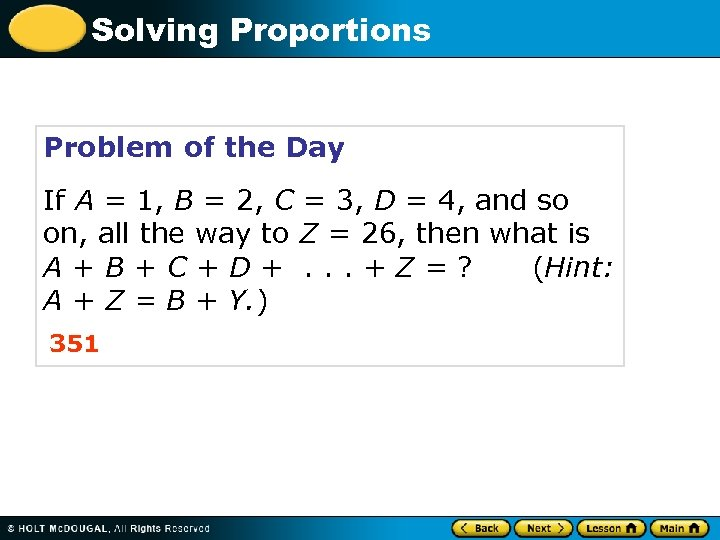Solving Proportions Problem of the Day If A = 1, B = 2, C = 3, D = 4, and so on, all the way to Z = 26, then what is A+B+C+D+. . . +Z=? (Hint: A + Z = B + Y. ) 351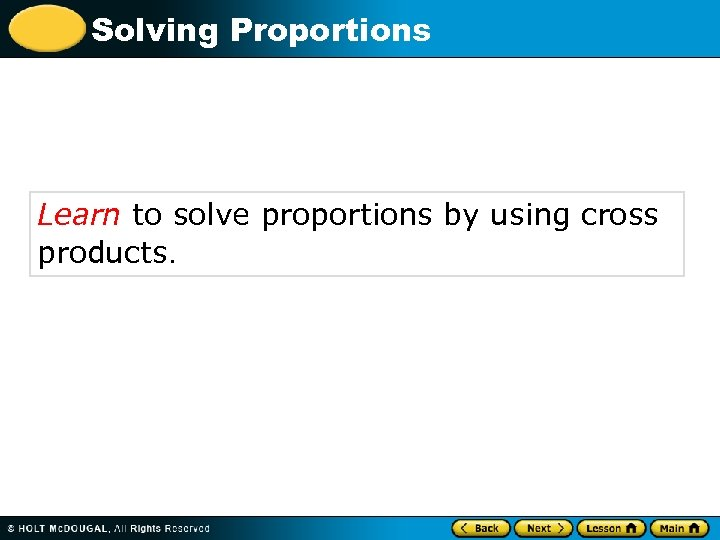Solving Proportions Learn to solve proportions by using cross products.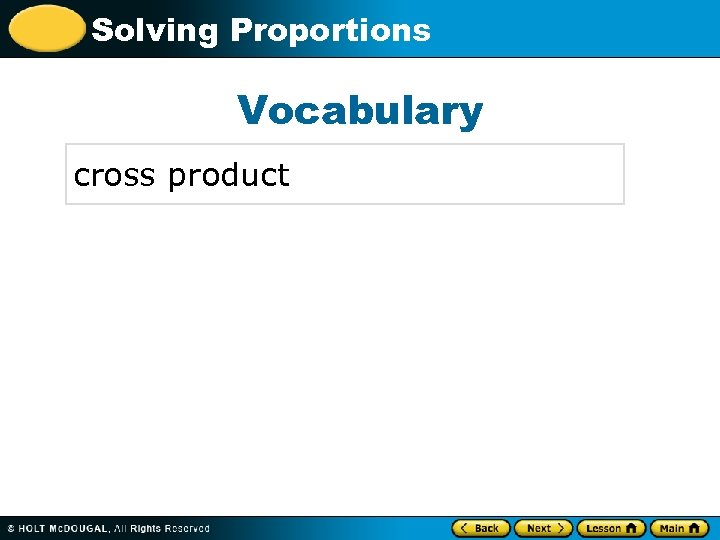Solving Proportions Vocabulary cross product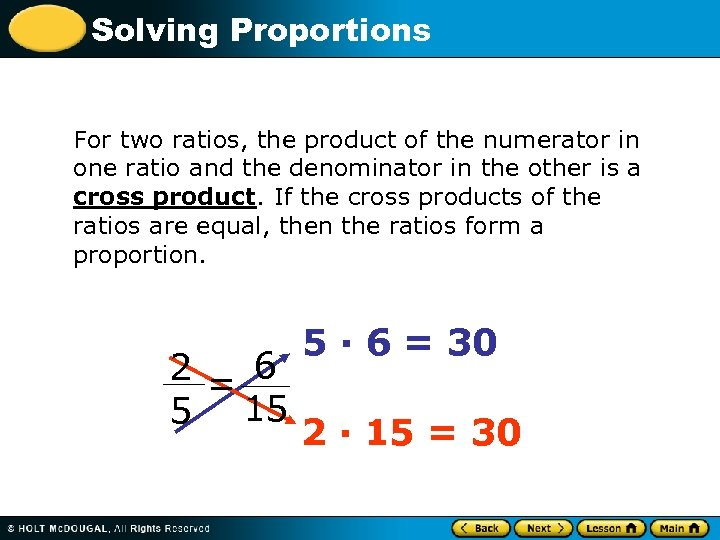Solving Proportions For two ratios, the product of the numerator in one ratio and the denominator in the other is a cross product. If the cross products of the ratios are equal, then the ratios form a proportion. 2 = 6 5 15 5 · 6 = 30 2 · 15 = 30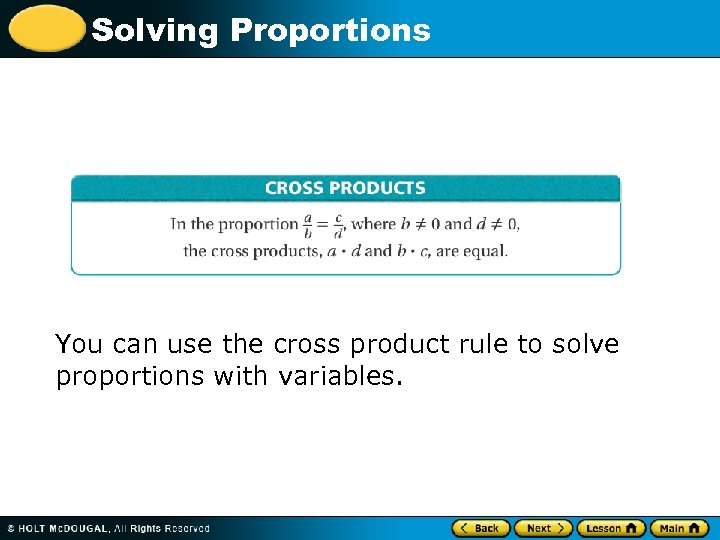Solving Proportions You can use the cross product rule to solve proportions with variables.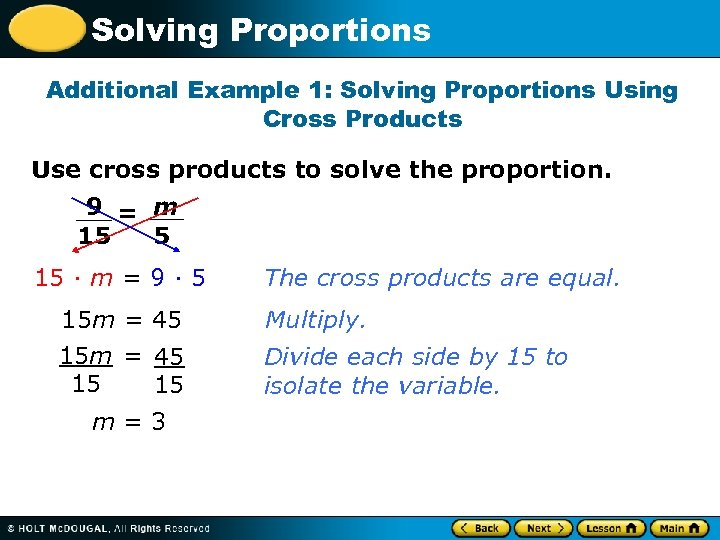Solving Proportions Additional Example 1: Solving Proportions Using Cross Products Use cross products to solve the proportion. 9 = m 5 15 15 · m = 9 · 5 15 m = 45 15 15 m=3 The cross products are equal. Multiply. Divide each side by 15 to isolate the variable.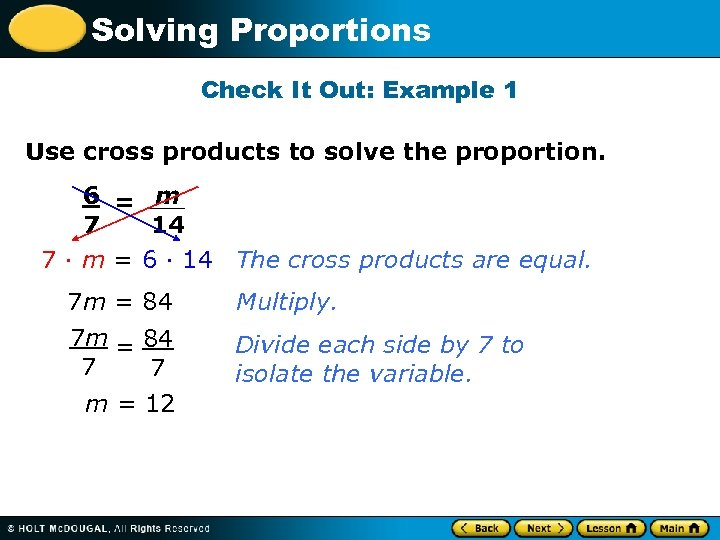Solving Proportions Check It Out: Example 1 Use cross products to solve the proportion. 6 = m 7 14 7 · m = 6 · 14 The cross products are equal. 7 m = 84 Multiply. 7 m = 84 7 7 m = 12 Divide each side by 7 to isolate the variable.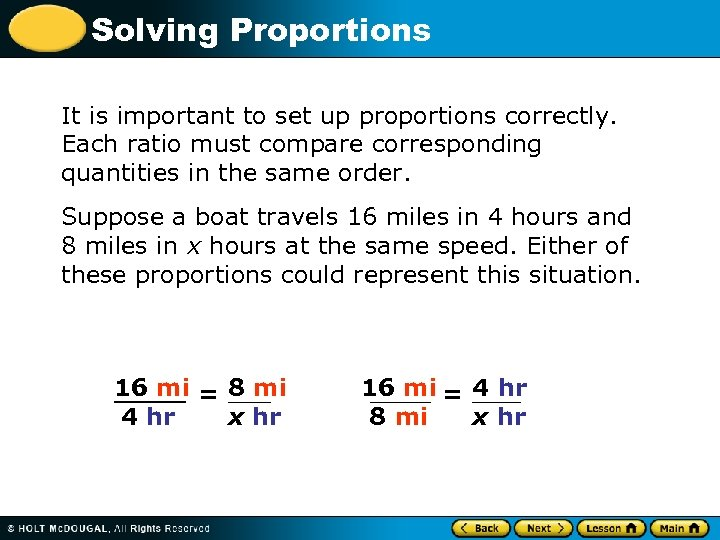Solving Proportions It is important to set up proportions correctly. Each ratio must compare corresponding quantities in the same order. Suppose a boat travels 16 miles in 4 hours and 8 miles in x hours at the same speed. Either of these proportions could represent this situation. 16 mi = 8 mi 4 hr x hr 16 mi = 4 hr 8 mi x hr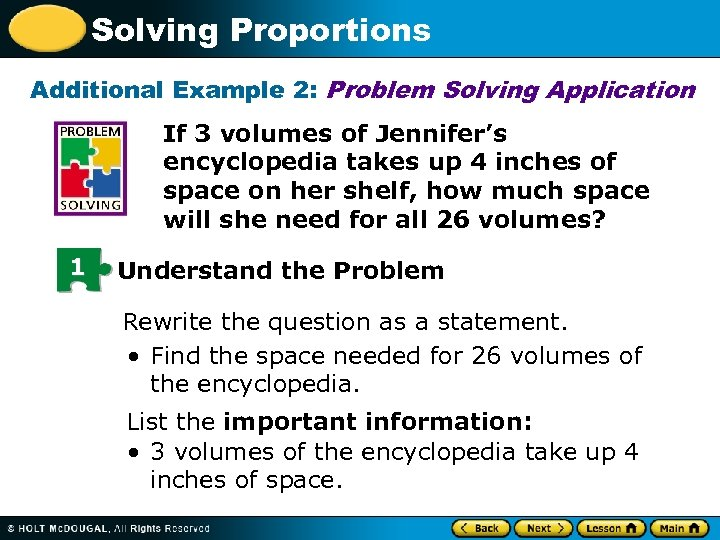Solving Proportions Additional Example 2: Problem Solving Application If 3 volumes of Jennifer’s encyclopedia takes up 4 inches of space on her shelf, how much space will she need for all 26 volumes? 1 Understand the Problem Rewrite the question as a statement. • Find the space needed for 26 volumes of the encyclopedia. List the important information: • 3 volumes of the encyclopedia take up 4 inches of space.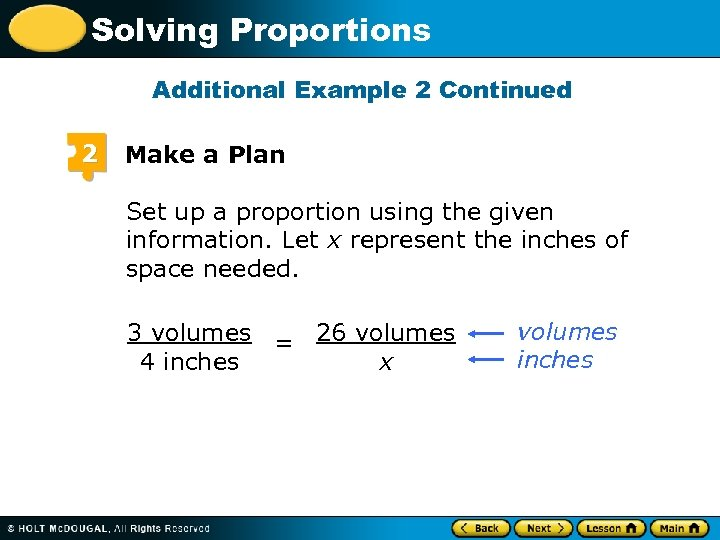Solving Proportions Additional Example 2 Continued 2 Make a Plan Set up a proportion using the given information. Let x represent the inches of space needed. 3 volumes = 26 volumes 4 inches x volumes inches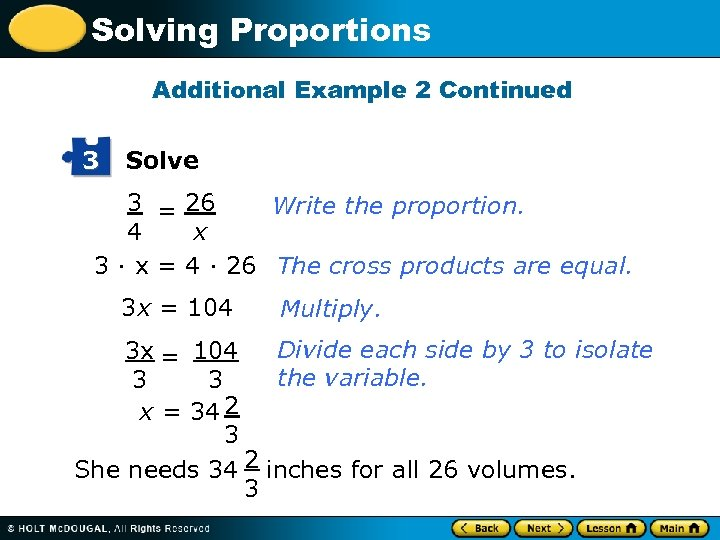Solving Proportions Additional Example 2 Continued 3 Solve 3 = 26 Write the proportion. 4 x 3 · x = 4 · 26 The cross products are equal. 3 x = 104 Multiply. Divide each side by 3 to isolate 3 x = 104 the variable. 3 3 x = 34 2 3 She needs 34 2 inches for all 26 volumes. 3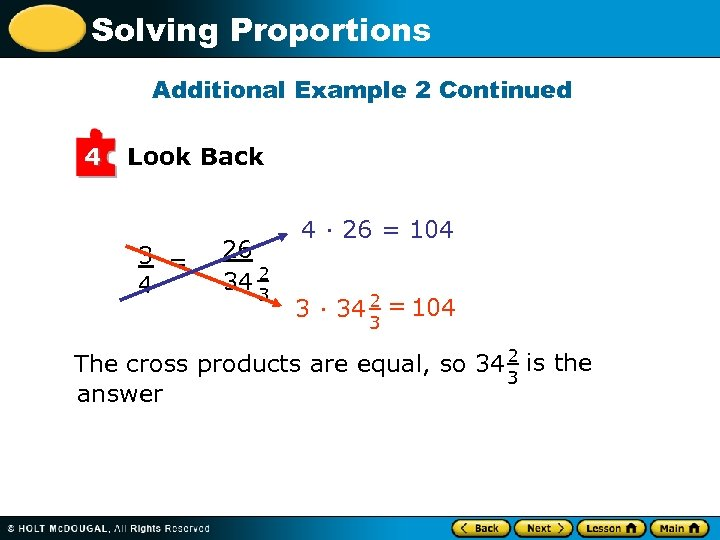Solving Proportions Additional Example 2 Continued 4 Look Back 3 = 4 26 34 2 3 4 · 26 = 104 3 · 34 2 = 104 3 The cross products are equal, so 34 2 is the 3 answer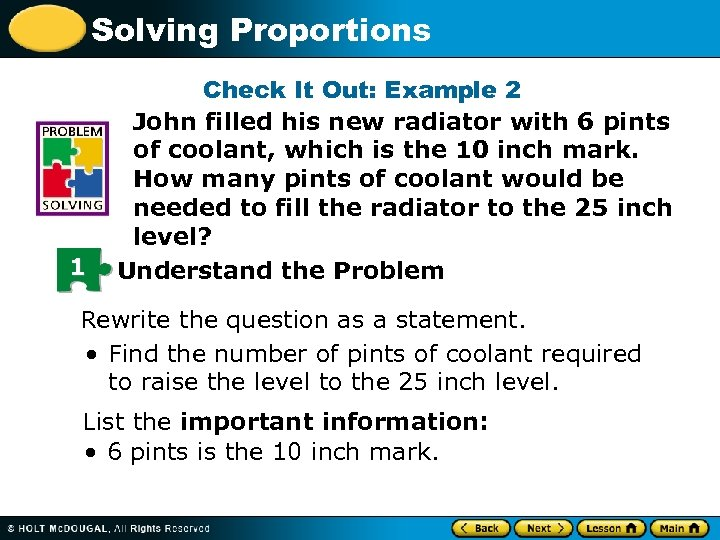Solving Proportions 1 Check It Out: Example 2 John filled his new radiator with 6 pints of coolant, which is the 10 inch mark. How many pints of coolant would be needed to fill the radiator to the 25 inch level? Understand the Problem Rewrite the question as a statement. • Find the number of pints of coolant required to raise the level to the 25 inch level. List the important information: • 6 pints is the 10 inch mark.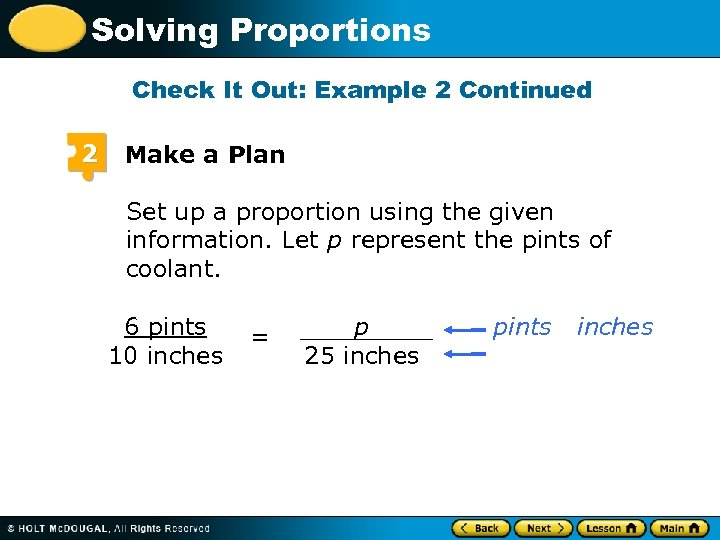Solving Proportions Check It Out: Example 2 Continued 2 Make a Plan Set up a proportion using the given information. Let p represent the pints of coolant. 6 pints 10 inches = p 25 inches pints inches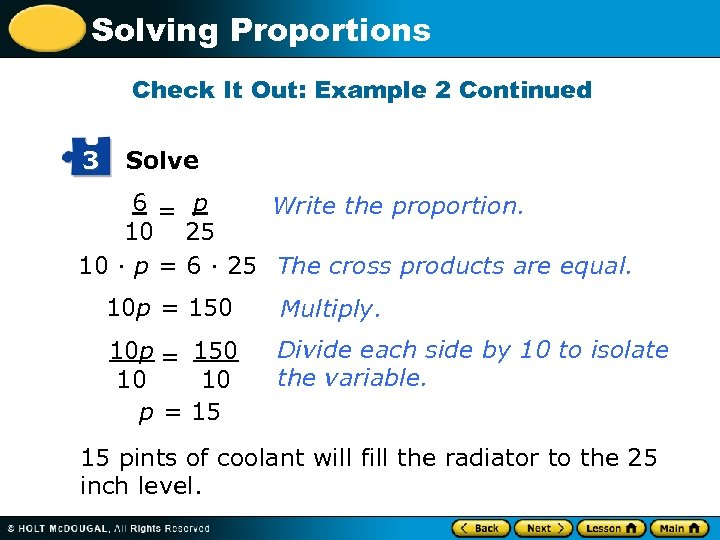Solving Proportions Check It Out: Example 2 Continued 3 Solve 6 = p Write the proportion. 10 25 10 · p = 6 · 25 The cross products are equal. 10 p = 150 Multiply. 10 p = 150 10 10 p = 15 Divide each side by 10 to isolate the variable. 15 pints of coolant will fill the radiator to the 25 inch level.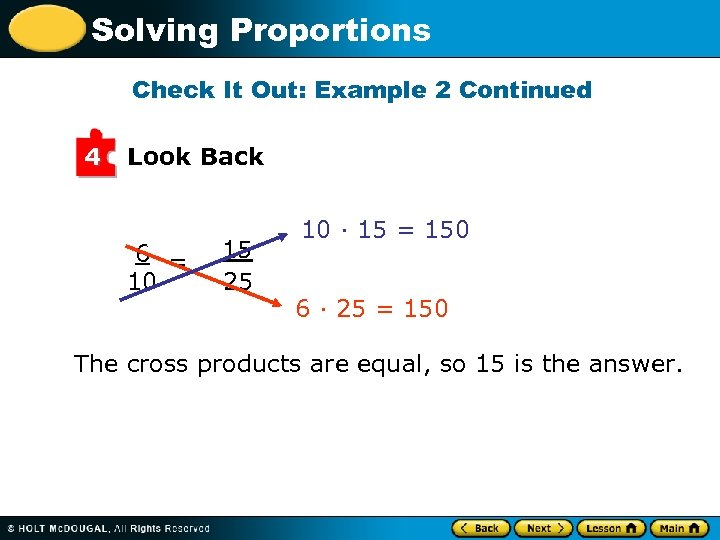Solving Proportions Check It Out: Example 2 Continued 4 Look Back 6 = 10 15 25 10 · 15 = 150 6 · 25 = 150 The cross products are equal, so 15 is the answer.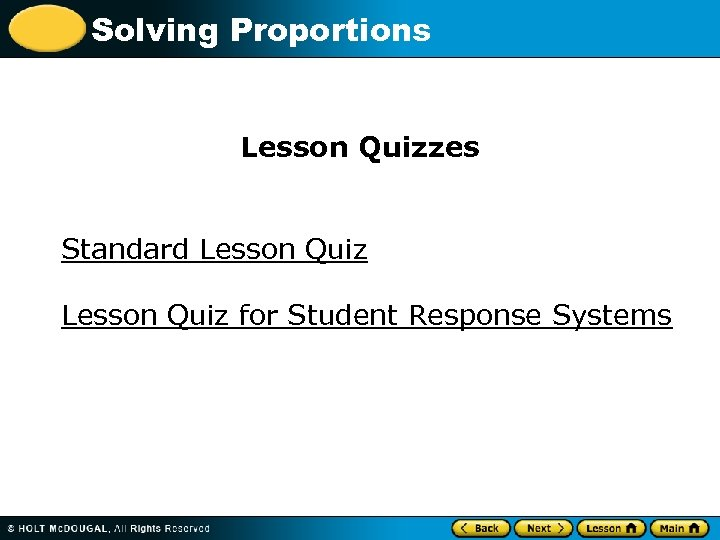Solving Proportions Lesson Quizzes Standard Lesson Quiz for Student Response Systems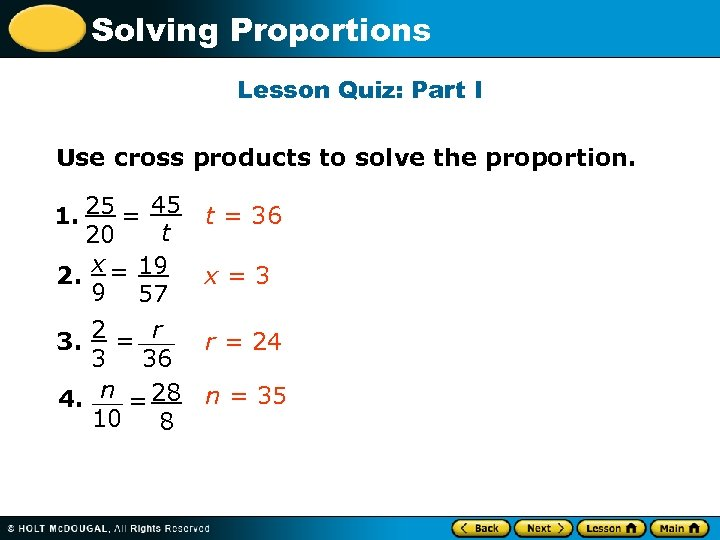Solving Proportions Lesson Quiz: Part I Use cross products to solve the proportion. 1. 25 = 45 t 20 2. x = 19 9 57 3. 2 = r 3 36 4. n = 28 10 8 t = 36 x=3 r = 24 n = 35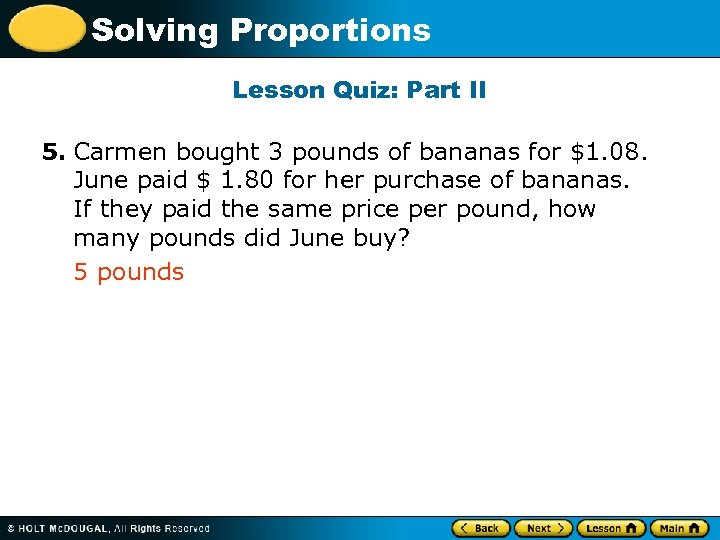Solving Proportions Lesson Quiz: Part II 5. Carmen bought 3 pounds of bananas for \$1. 08. June paid \$ 1. 80 for her purchase of bananas. If they paid the same price per pound, how many pounds did June buy? 5 pounds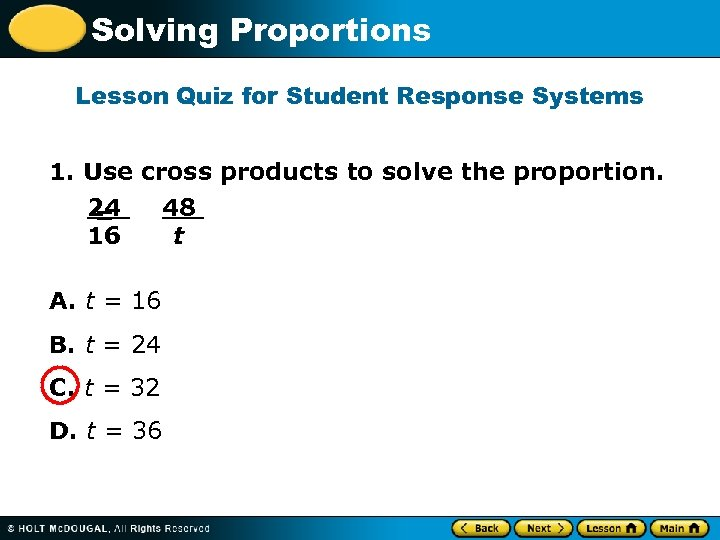Solving Proportions Lesson Quiz for Student Response Systems 1. Use cross products to solve the proportion. 24 48 = 16 t A. t = 16 B. t = 24 C. t = 32 D. t = 36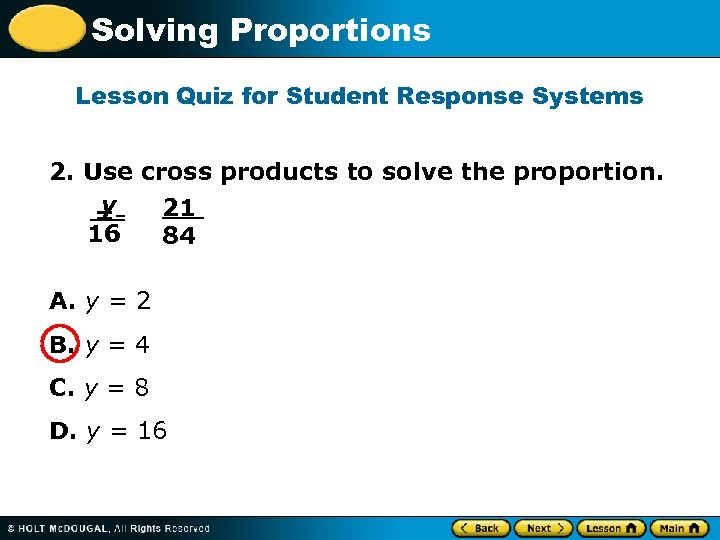Solving Proportions Lesson Quiz for Student Response Systems 2. Use cross products to solve the proportion. y 21 = 16 84 A. y = 2 B. y = 4 C. y = 8 D. y = 16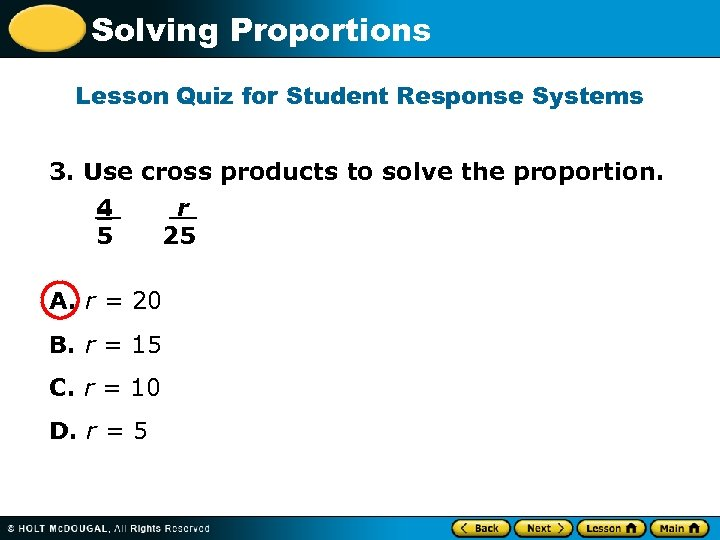Solving Proportions Lesson Quiz for Student Response Systems 3. Use cross products to solve the proportion. 4 r = 5 25 A. r = 20 B. r = 15 C. r = 10 D. r = 5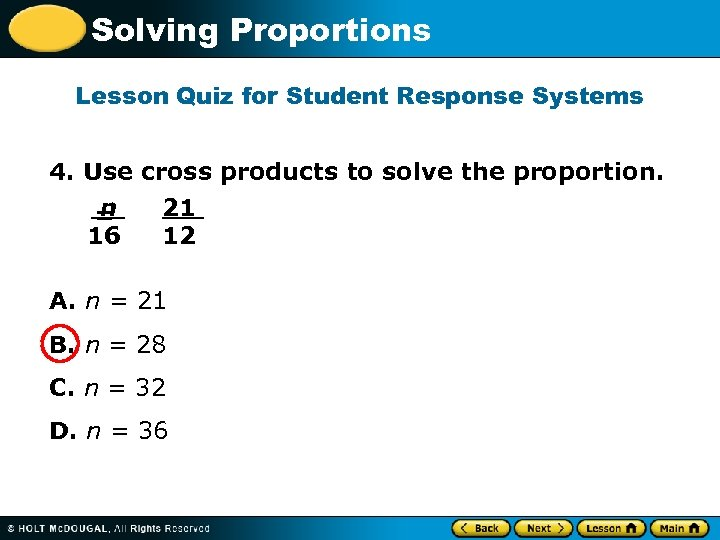Solving Proportions Lesson Quiz for Student Response Systems 4. Use cross products to solve the proportion. n 21 = 16 12 A. n = 21 B. n = 28 C. n = 32 D. n = 36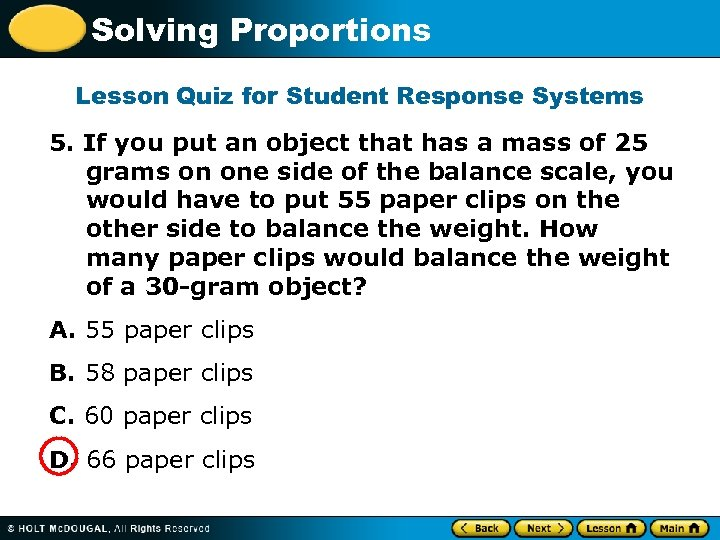Solving Proportions Lesson Quiz for Student Response Systems 5. If you put an object that has a mass of 25 grams on one side of the balance scale, you would have to put 55 paper clips on the other side to balance the weight. How many paper clips would balance the weight of a 30 -gram object? A. 55 paper clips B. 58 paper clips C. 60 paper clips D. 66 paper clips